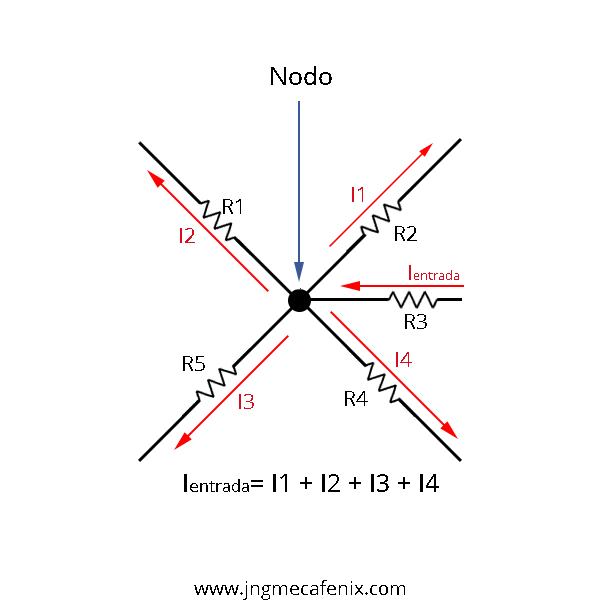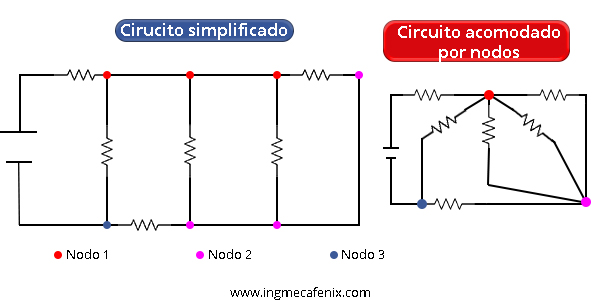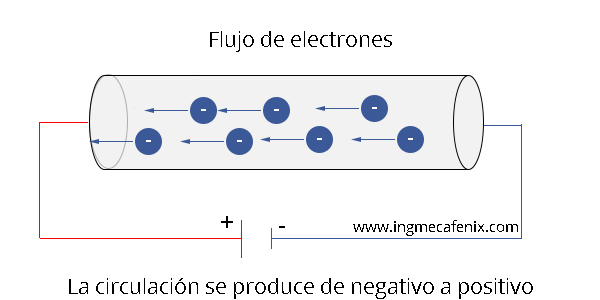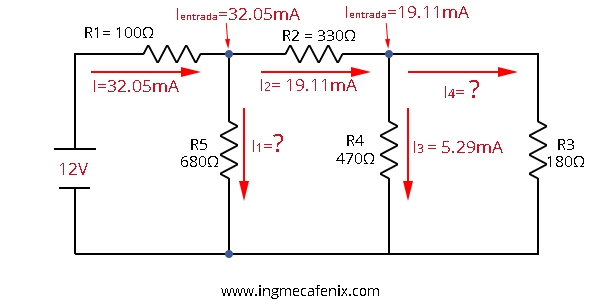# KIRCHHOFF’S CURRENT LAW# What does Kirchhoff’s current law say?

The current law or also known as Kirchhoff’s first law or node law (LCK) establishes that «At any node, the algebraic sum of the electric currents that enter is equal to the sum of currents that leave. As an equivalent, the algebraic sum of all the currents passing through the node is equal to zero »### Node

It is the union between two or more elements within an electrical circuit. As for the nodes, they can be seen with the naked eye or distributed along the same cable, but all of them are connected to the same cable.### Electric current

Electric current is a flow of electrons that circulates through conductive materials in a closed circuit.## Example

This law is fundamental within electrical circuits since it makes our work easier, for example when we know the incoming current at a node and we have the current data of each output except one we can apply the statement to quickly know what current value has output missing.

In this example we can see that we have 2 nested nodes and each one is made up of 1 input current (input) and two output ones. The data we have for each node is the input current and only one output, applying Kirchhoff’s current law we can easily know what the value of our unknowns is.To solve this exercise we simply have to clear the formula of this law and replace the values ​​and solve the equation to know the value of our unknown.